# 6.1 Wind calculation method

Numerical simulations of the wind around the Main Building were performed by the commercially available CFD package Fluent. We used versions 4.4 and 4.5 and the differences between these two versions are not relevant for our purpose. The used turbulence model is a standard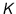-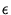model ([Fluent 1995], or see e.g. [Launder and Spalding 1974] and [Rodi 1980]).

Model constants The applied values for the-model constants are: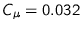,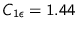,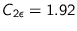,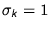and. Except for, the standard values of the-model have been applied (the model and the constants are described in [Launder and Spalding 1974]). Theconstant was adapted according to the findings of [Bottema 1993b], who also compared the results of his simulations favourably with wind tunnel measurements.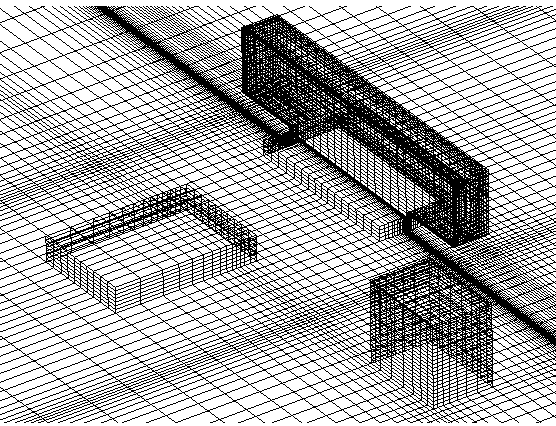Grid The applied grid is a so-called structured grid. The size of the three-dimensional computational domain was determined by the rules of thumb given by [Bottema 1993a] and based on general estimates of the influence zone in which wind speeds deviate by more than 10% due to the presence of the building. The reader is also referred to section 2.1.4. For the description of this zone the dimension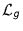, i.e. the smaller ofand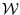, is used. The upstream influence zone is about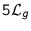and its downstream counterpart extends to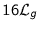. The influence zone in lateral and vertical directions extends to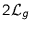. The boundaries of the computational domain should be outside the influence zone, although for the downstream influence zone one may make an exception and put the boundary at only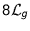[Bottema 1993a].

Asis 90 m (=) in our case, the computational domain should be about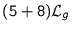(=1170 m) long along the wind direction. To be able to simulate wind flow around the Main Building at oblique angles, the chosen computational domain is rectangular with the Main Building situated in the south-west corner, atfrom the south and the west domain boundaries.

The actual three-dimensional computational domain is 1190 m long in east-west direction, 1477 m long in north-south direction and 225 m high. It consists of 95, 96 and 47 cells, respectively. Figure 6.1 shows the computational grid with the three buildings (Auditorium, Main Building and Building T) in it. Two variants of the shown configuration will be applied: with and without Building T. There are two reasons for this. Firstly, the influence of Building T on winds from south-west to south can be presented. Secondly, the grid without Building T is symmetric with respect to the east-west plane. This implies that winds from directions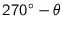and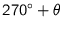(where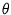is a value from 0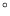to 90) can be studied using one simulation.

The grid cells become progressively smaller near building boundaries. The first grid cells on the façade of the Main Building have a thickness of 0.25 m. Care is taken to keep the grid expansion factor of two successive grid lines between 0.7 and 1.3. However, since the grid is structured, undesired large expansion factors and cell aspect ratios are inevitable in some parts of the grid.

Wind profile The profile of the wind entering the domain is described by: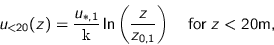(6.1)

and: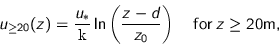(6.2)

with= longitudinal wind velocity [m s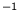] at height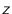[m] above ground level,= roughness length [m] for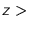20 m,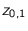= roughness length [m] for20 m,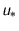= friction velocity [m s],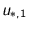= friction velocity [m s], resulting from the requirement thatis continuous at20 m,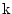= von Kármán constant (0.4), and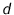= displacement height [m].

The applied values of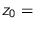1.0 m and10 m were taken from results of measurements at the site by [Geurts 1997]. The division of the wind profile in two parts is necessary to account for the displacement heightof 10 m; otherwise, the wind profile below 10 m height would be undetermined. Moreover, the fetch consists of a park up to a distance of 400 m from the Main Building (therefore an estimatedof 0.1 m) and buildings west from the park with a height of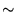20 m. The choice ofand the choice that the boundary between the two parts of the profile is at 20 m height, are relatively arbitrary, but the simulation results are not very sensitive to the precise values of these parameters.

The friction velocityis based on the wind speed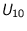at Eindhoven Airport (7.5 km westward from the Main Building, with= 10 m,= 0.03 m and= 0 m). See section 5.2.1 for a discussion on the measurements of.

Turbulent kinetic energy The profiles of the turbulent kinetic energy and its dissipation rate, for wind coming into the domain are described by:(6.3)

and:(6.4)

respectively, with= turbulent kinetic energy per unit of mass [m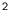s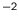], and= energy dissipation rate [ms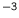].

Terrain roughness In Fluent the roughness of surfaces is modelled by the following formula: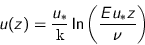(6.5)

where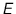is a roughness parameter [-] andis the kinematic viscosity [ms] of air.

The roughness parameter is empirically determined. Its value is 9.8 for a smooth wall. Equation 6.5 corresponds to the wind profile (eqs. 6.1 and 6.2) if: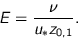(6.6)

Façade roughness Equations 6.5 and 6.6 are also applied to model the surface roughness of the building façades. As the façade consists of a smooth glass cladding, a value of 0.0005 m is assumed for its roughness length,

Separation modelling Separation of the airflow at corners has been modelled by so-called link-cuts'' (i.e. a feature of Fluent by which the wall-function in a computational cell is disabled).

Chosen wind speeds and directions The following reference wind speeds and directions at the mast on the Auditorium were chosen for the wind calculations:

•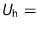3.5, 5.7 and 11.2 m s,
•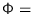210, 240, 270, 300and 330.

Not every combination was simulated, see table 6.2 for the simulations which were actually performed. Recall that we use two geometrical models, namely with and without the inclusion of Building T, and because of symmetry, the simulations with240will be used to represent300too. The validity of these `double simulations' will be discussed in section 6.3.

© 2002 Fabien J.R. van Mook
ISBN 90-6814-569-X
Published as issue 69 in the Bouwstenen series of the Faculty of Architecture, Planning and Building of the Eindhoven University of Technology.